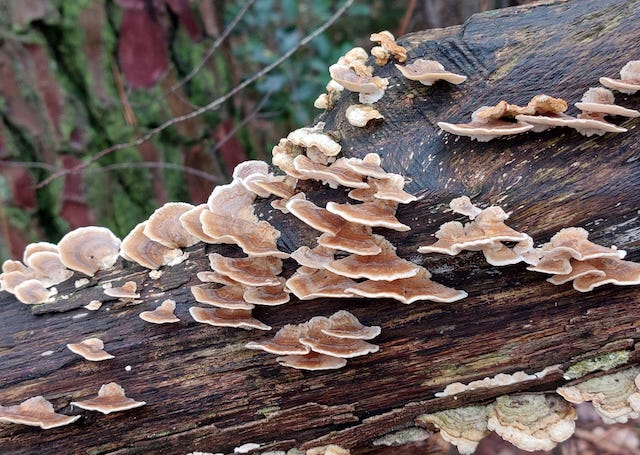# Decision Trees

This category groups articles that are oriented on the topic of Decision Trees. Each post focuses on specific aspects, or attributes, of Decision Trees. The emphasis here is on gaining a deep intuition for how Decision Trees work. Worked examples in Python are provided.## Can Decision Trees Handle Categorical Features?

Can Decision Trees Handle Categorical Features? Yes, Decision Trees handle categorical features naturally. Often these features are treated by first one-hot-encoding (OHE) in a preprocessing step. However, it is straightforward to extend the CART algorithm to make use of categorical features without such preprocessing. In this post, I will implement classification and regression Decision Trees capable …## Can Decision Trees Handle Missing Values?

Can Decision Trees Handle Missing Values? Yes, Decision Trees handle missing values naturally. It is straightforward to extend the CART algorithm to support the handling of missing values. However, attention needs to be made regarding how the algorithm is implemented in code. In this post, I will implement classification and regression Decision Trees capable of dealing …## Are Decision Trees Robust to Outliers?

Are Decision Trees Robust to Outliers? In general, Decision Trees are quite robust to the presence of outliers in the data. This is true for both training and prediction. However, care needs to be taken to ensure the Decision Tree has been adequately regularised. An overfitted Decision Tree will show sensitivity to outliers. Why are …## How to Interpret Decision Trees with 1 Simple Example

How to Interpret Decision Trees with 1 Simple Example We can interpret Decision Trees as a sequence of simple questions for our data, with yes/no answers. One starts at the root node, where the first question is asked. Based upon the answer, we navigate to one of two child nodes. Each child node asks an additional …## 3 Methods to Tune Hyperparameters in Decision Trees

3 Methods to Tune Hyperparameters in Decision Trees We can tune hyperparameters in Decision Trees by comparing models trained with different parameter configurations, on the same data. An optimal model can then be selected from the various different attempts, using any relevant metrics. There are several different techniques for accomplishing this task. Three of the …## How to Measure Information Gain in Decision Trees

How to Measure Information Gain in Decision Trees For classification problems, information gain in Decision Trees is measured using the Shannon Entropy. The amount of entropy can be calculated for any given node in the tree, along with its two child nodes. The difference between the amount of entropy in the parent node, and the …